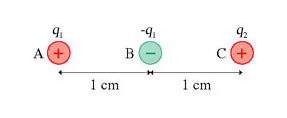# Problem: What is the magnitude of the net electric force on charge A in the figure (Figure 1)? Assume that q1 = 1.5nC and q2 = 4.8nC.

###### FREE Expert Solution

Coulomb's law:

$\overline{){\mathbf{F}}{\mathbf{=}}\frac{\mathbf{k}{\mathbf{q}}_{\mathbf{1}}{\mathbf{q}}_{\mathbf{2}}}{{\mathbf{r}}^{\mathbf{2}}}}$

90% (107 ratings)###### Problem Details

What is the magnitude of the net electric force on charge A in the figure (Figure 1)? Assume that q1 = 1.5nC and q2 = 4.8nC.